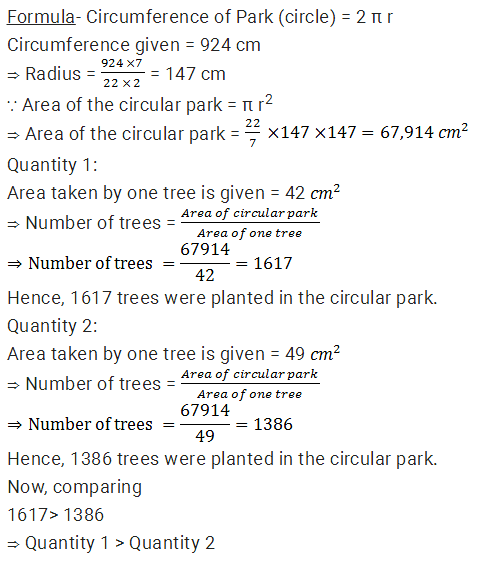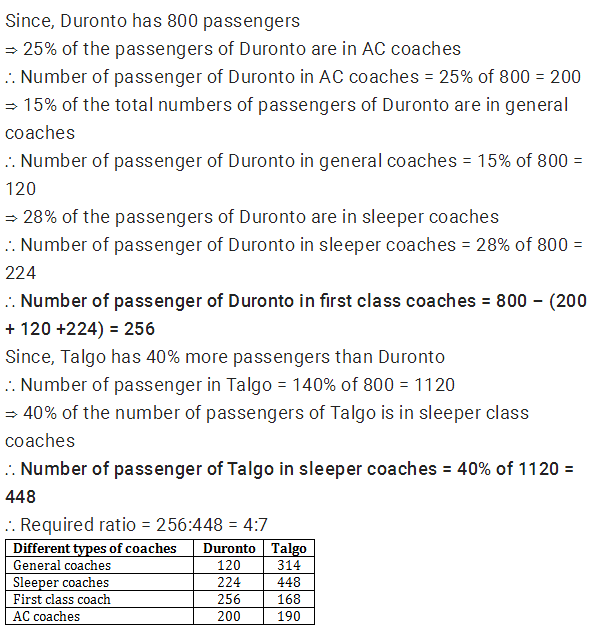## Quantitative Aptitude Practice Questions for SBI PO 2017

Quantitative Aptitude Practice Questions for SBI PO 2017
Directions (1 – 5): Find the appropriate relation for quantity1 and quantity2 in the following question:
1. Quantity 1: In an examination, Ankita scored 35 marks less than Puneeta. Puneeta scored 65 more marks than Meenakshi. Rakhi scored 115 marks, which is 20 marks more than Meenakshi's. Simpy scored 108 marks less than the maximum marks of the test. What approximate percentage of marks did Simpy score in the examination, if she got 67 marks more than Ankita?
Quantity 2: The length of a rectangle is increased by 60%. By what percent would the width have to be decreased to maintain the same area?

a) Q1 < Q2
b) Q1 ≤ Q2
c) Q1 ≥ Q2
d) Q1 > Q2
e) None of these
2. A bag contains 20 balls which include 7 balls of red colour, 8 balls of white colour and rest of green colour. If 7 balls are drawn then probability of
Quantity 1:3 balls of red colour and 4 balls of white colour
Quantity 2:5 balls of white colour and 2 balls of green colour
a) Q1 > Q2
b) Q1 < Q2
c) Q1 ≥ Q2
d) Q1 ≤ Q2
e) Q1 = Q2 or no relationship exits
3. Quantity 1: 91854, 10,206, 1,134, 126, (?)
Quantity 2: 38, 43, 53, (?), 113, 193
a) Q1 > Q2
b) Q1 < Q2
c) Q1 ≥ Q2
d) Q1 ≤ Q2
e) Q1 = Q2 or no relationship exits
4. How many trees can be put in Circular Park whose circumference is 924cm
Quantity 1: allowing 42cm2 for each tree
Quantity 2: allowing 49cm2 for each tree
a) Q1 > Q2
b) Q1 < Q2
c) Q1 ≥ Q2
d) Q1 ≤ Q2
e) Q1 = Q2 or no relationship exits
5. Quantity 1: Number of hours in which A, B and C can complete the work if A, B and C alone can finish it in 25, 20 and 15 hours respectively.
Quantity 2: Number of hours in which B can finish the work if A working alone finishes it in 20 hours while working together they finish it in 5 hours.
a) Q1 > Q2
b) Q1 ≥ Q2
c) Q1 ≤ Q2
d) Q1 > Q2
e) Q1 = Q2
Directions (6 – 10): Study the following information carefully to answer the questions that follow.
There are two trains Duronto and Talgo. Both trains have four different types of coaches i.e general coaches, sleeper coaches, first class coaches and AC coaches. In Duronto there are 800 passengers. Talgo has 40% more passengers than Duronto. 25% of the passengers of Duronto are in AC coaches. 15% of the total numbers of passengers of Duronto are in general coaches, 28% of the passengers of Duronto are in sleeper coaches and remaining passengers of Duronto are in first class coaches. 40% of the numbers of passengers of Talgo are in sleeper class coaches. Total number of passengers in AC coaches in both the train together is 390 and 15% of total passenger of Talgo are in first class coach. Remaining passengers of Talgo are in general class coach.
6. What is the ratio of the number of passengers in first class coaches of Duronto to the number of passengers in sleeper class coaches of Talgo?
a) 3 : 7
b) 17 : 13
c) 2 : 3
d) 4 : 7
e) None of these
7. What is the total number of passengers in the general coaches of Duronto and Talgo together?
a) 449
b) 459
c) 434
d) 445
e) None of these
8. What is the difference between the number of passengers in the AC coaches of Duronto and total number of passengers in sleeper class coaches and AC coaches together of Talgo?
a) 300
b) 438
c)  487
d) 279
e) None of these
9. Total number of passengers in First class coaches in both the trains together is approximately what percentage of total number of passengers in Talgo?
a) 35
b) 42
c) 45
d) 38
e) 31
10. If cost of per ticket of AC coach of both the train is Rs.350, what total amount will be generated from AC coaches of both the train?
a) Rs.136500
b) Rs.108000
c) Rs.111200
d) Rs.145600
e) None of these
Solutions:
1. D) Quantity 1-
Rakhi’s marks= 115
Meenakshi’s marks= 115 - 20 = 95
Puneeta’s marks= 95 + 65= 160
Ankita’s marks=160 - 35= 125
Simpy’s marks= 125+ 67= 192
Total maximum marks= 192 + 108= 300
Required percentage marks of Simply =  192/300 × 100 = 64%
Quantity 2-
Let length and breadth be 100.
After increase in length it become 160, then reduction in breadth be ‘x’
Now, 160*x= 100*100
Hence, x =  10000/160 = 62.5
Reduction in breadth is 100 – 62.5 = 37.5%
Hence, Quantity1 > Quantity2
2. A)3. B)4. A)5. D)6. D)7. C)
Number of passenger of Duronto in general coaches = 15% of 800 = 120
Number of passenger of Talgo in general coaches = 1120 – (448 + 190 + 168) = 314
Total number of passengers in the general coaches of Duronto and Talgo = 120 + 314 = 434
8. B)
Number of passenger of Talgo in sleeper coaches = 40% of 1120 = 448
Number of passenger of Talgo in AC coaches = 390 – 200 = 190
Require difference = (448 + 190) – 200 = 438
9. D)
Number of passenger of Duronto in first class coaches = 800 (200 + 120 +224) = 256
Number of passenger in Talgo = 140% of 800 = 1120
Number of passenger of Talgo in first class coach = 15% of 1120 = 168
Required percentage = (256 + 168)/1120 × 100 = 37.85% ≈ 38%
10. A)
Total number of passengers in AC coaches in both the train together is 390
Amount generated from AC coaches of both the train = 390 × 350 = Rs. 136500